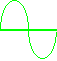e Ham Radio ~ Ham ToolsRadio  CalculatorEnter Results ^ Enter value then click a function key - * 50 Ohm Conversions Copyright © Charlie, W5AM (Radioing.com).

 Calculator Conversion Units Note:  Conversions marked with (*) are for 50 ohm calculations only. dB = decibel, (10 Log10P) P = Power Ratio, (P2/P1) u = micro = 10E-6 m = milli = 10E-3 dBw = decibels relative to one watt dBm = decibels relative to one milliwatt dBV = decibels relative to one volt dBuV = decibels relative to one microvolt dBuA = decibels relative to one microamp V = Volts Vpp = Volts, AC peak-to-peak Vrms = Volts, AC root-mean-square (rms) A = Amps R = Ohms (50 ohms) W = Watts